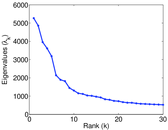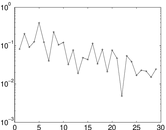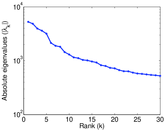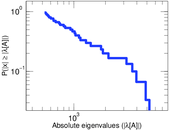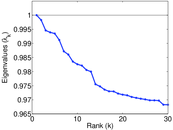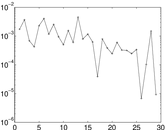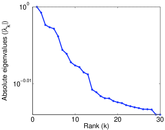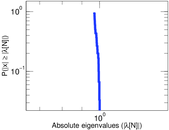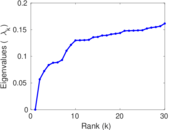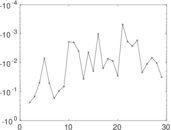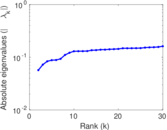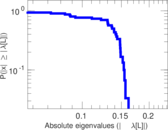# Wikiquote edits (en)

This is the bipartite edit network of the English Wikiquote. It contains users and pages from the English Wikiquote, connected by edit events. Each edge represents an edit. The dataset includes the timestamp of each edit.

 Code `qen` Internal name `edit-enwikiquote` Name Wikiquote edits (en) Data source http://dumps.wikimedia.org/ AvailabilityDataset is available for download Consistency checkDataset passed all tests Category Authorship network Dataset timestamp 2017-10-20 Node meaning User, article Edge meaning Edit Network formatBipartite, undirected Edge typeUnweighted, multiple edges Temporal dataEdges are annotated with timestamps

## Statistics

 Size n = 186,686 Left size n1 = 35,962 Right size n2 = 150,724 Volume m = 1,271,653 Unique edge count m̿ = 482,024 Wedge count s = 2,171,858,958 Claw count z = 13,483,634,713,119 Cross count x = 70,752,413,484,809,184 Square count q = 940,627,482 4-Tour count T4 = 16,213,772,192 Maximum degree dmax = 125,310 Maximum left degree d1max = 125,310 Maximum right degree d2max = 10,169 Average degree d = 13.623 4 Average left degree d1 = 35.361 0 Average right degree d2 = 8.436 96 Fill p = 8.892 88 × 10−5 Average edge multiplicity m̃ = 2.638 15 Size of LCC N = 179,403 Diameter δ = 12 50-Percentile effective diameter δ0.5 = 3.403 16 90-Percentile effective diameter δ0.9 = 4.379 51 Median distance δM = 4 Mean distance δm = 3.803 27 Gini coefficient G = 0.860 083 Balanced inequality ratio P = 0.140 007 Left balanced inequality ratio P1 = 0.081 596 9 Right balanced inequality ratio P2 = 0.181 053 Relative edge distribution entropy Her = 0.759 277 Power law exponent γ = 2.668 43 Tail power law exponent γt = 2.471 00 Tail power law exponent with p γ3 = 2.471 00 p-value p = 0.000 00 Left tail power law exponent with p γ3,1 = 2.031 00 Left p-value p1 = 0.000 00 Right tail power law exponent with p γ3,2 = 3.051 00 Right p-value p2 = 0.002 000 00 Degree assortativity ρ = −0.233 375 Degree assortativity p-value pρ = 0.000 00 Spectral norm α = 5,268.11 Algebraic connectivity a = 0.056 802 1 Spectral separation |λ1[A] / λ2[A]| = 1.084 48 Controllability C = 142,784 Relative controllability Cr = 0.773 591

## Plots

### Fruchterman–Reingold graph drawing### Degree distribution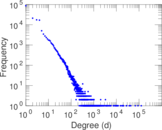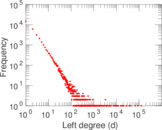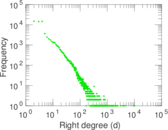### Cumulative degree distribution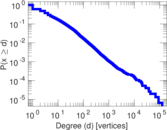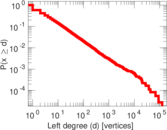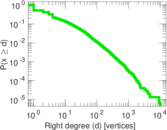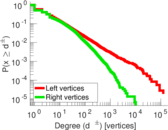### Lorenz curve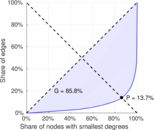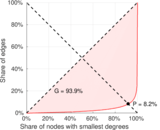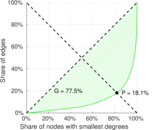### Spectral distribution of the adjacency matrix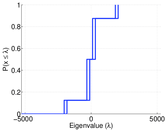### Spectral distribution of the normalized adjacency matrix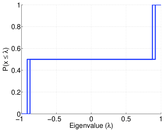### Spectral distribution of the Laplacian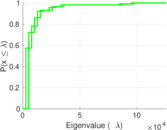### Spectral graph drawing based on the adjacency matrix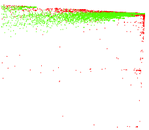### Spectral graph drawing based on the Laplacian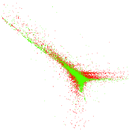### Spectral graph drawing based on the normalized adjacency matrix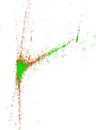### Degree assortativity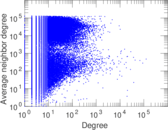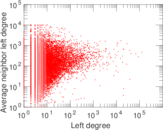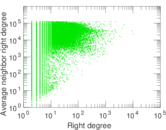### Zipf plot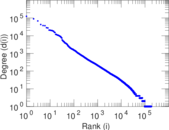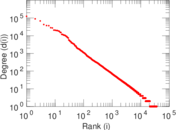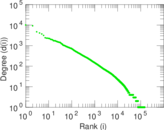### Hop distribution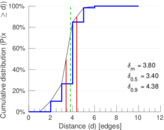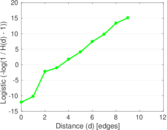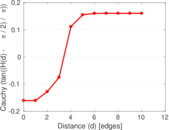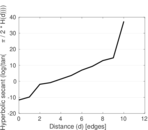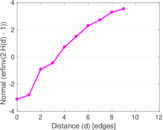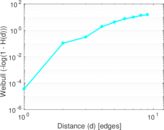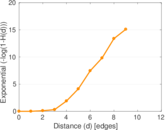### Delaunay graph drawing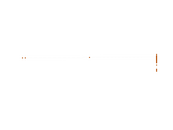### Edge weight/multiplicity distribution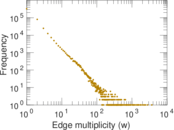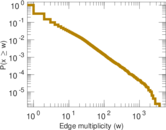### Temporal distribution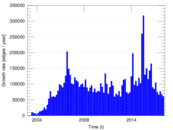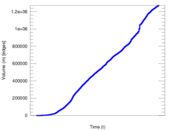### Temporal hop distribution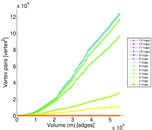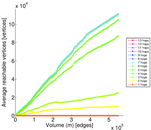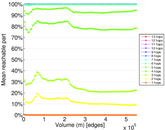### Diameter/density evolution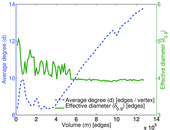### Inter-event distribution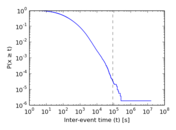### Node-level inter-event distribution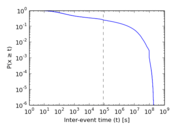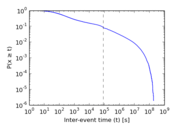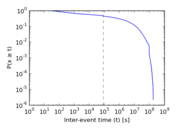### Matrix decompositions plots